# Calculating and visualizing Gimbal angles.

#### Mick West

Staff member
Thank you very much Mick! Although in the end the explanation proves that there is no other parameter that needs to be added to your beautiful tool, this one necessarily had to be done. Can you show me the code of this latest addition? I can better understand the behavior through.... numbers

This is the code (freshly cleaned and commented) that takes an Az value and converts it into CueAz (the angle of the Cue dot).
JavaScript:
``````// Take a frame number in the video (i.e. a time in 1/30ths)
// and return the angle formed by projecting the camera's Az/El vector
// onto the plane of the wings
function Frame2CueAz(frame) {

// get az for this frame (el is constant, in par.el)
// this comes from video data, shown on the graph as yellow
var az = Frame2Az(frame)

// get a unit vector in the direction of Az/El
// the Az=0 and El=0 is always along the -Z axis
// This can be thought of as a position on a unit sphere.
// El is relative to the horizontal plane, so AoA is irrelevant
// Az is relative to the jet's forward direction in the horizontal plane
var AzElHeading = EA2XYZ(par.el, az, 1)

// Create a Plane object, representing the wing plane
// (a "plane" is a flat 2D surface in 3D space, not an aeroplane)
// the plane in Hessian normal form, normal unit vector (jetUp)
// and a distance from the origin (0, as the origin is the ATFLIR camera, i.e. the jet)
var jetRoll = jetRollFromFrame(frame) // get jet roll angle from either video data or constant
var jetUp = new THREE.Vector3(0, 1, 0) // y=1 is the jet up unit vector with no rotation
jetUp.applyAxisAngle(V3(0,0,1),-radians(jetRoll)) // apply roll about Z axis (-Z is fwd, so -ve)
var wingPlane = new THREE.Plane(jetUp,0)

// now find the jet's forward vector, which will be in the wing plane
// same rotations as with the up vector
var jetForward = new THREE.Vector3(0, 0, -1)

// calculate the angle between the jet forward vector

// angleTo always returns a positive value, so we
// need to negate it unless cross product is in same direction as jet up
// the cross product will give a vector either up or down from the plane
// depending on if cueHeading is left or right of JetForward when looking down
// then use a dot product which returns positive if two vectors are in the same direction
var sign = cross.dot(jetUp)
if (sign < 0) cueAz = -cueAz

// The return value is plotted in cyan (light blue)
return cueAz
}``````

And the code that makes the arrows
JavaScript:
``````//  var rotationMatrix = new THREE.Matrix4().extractRotation(PodFrame.matrixWorld);
//  var jetUp = new THREE.Vector3(0, 1, 0).applyMatrix4(rotationMatrix).normalize();

// The rotations below are equivalent to the above,
// but I'm doing it explicitly like this
// to match the code in Frame2CueAz,
// so the graph uses the same code as the arrows
// except instead of using a unit sphere
// to get the large arrows in the display.
var jetUp = new THREE.Vector3(0, 1, 0)
var jetPlane = new THREE.Plane(jetUp,0) // plane in Hessian normal form, normal unit vector and a distance from the origin
// take the idealPos (the white dot) and project it onto the jetPlane
var cuePos=new THREE.Vector3;
jetPlane.projectPoint(idealPos,cuePos) // project idealPos onto jetPlane, return in cuePos
DebugArrowAB("Projected Cue",idealPos,cuePos,0x00ffff,false)
DebugArrowAB("Cue Az",V3(0,0,0),cuePos,0x00ffff,false)

var horizonPlane = new THREE.Plane(V3(0,1,0),0)
var azPos=new THREE.Vector3;
horizonPlane.projectPoint(idealPos,azPos) // the same as just setting y to 0
DebugArrowAB("Projected Az",idealPos,azPos,0xffff00,false)
DebugArrowAB("Az",V3(0,0,0),azPos,0xffff00,false)``````

You can also just view source with the tool itself. https://www.metabunk.org/gimbal/

#### Mick West

Staff member
I've deleted a large post by @dimebag2, as it's substantially off topic for this thread which is focussed on "calculating and visualizing gimbal angles", not on things like calculating probabilities of planes being in particular positions.

I do not do this lightly. Experience has show that allowing a wide variety of discussion topics in a complex subject results in massive thread drift, and the obscuration of the actual subject of the thread (see the 100 page 9/11 threads for example).

#### Leonardo Cuellar

##### Active Member
This is the code (freshly cleaned and commented) that takes an Az value and converts it into CueAz (the angle of the Cue dot).
Thanks again Mick. Observing the code and playing a bit on the tool, the cue dot is also a function of the elevation. And AOA and elevation are directly proportional. Very interesting.

#### Mick West

Staff member
Thanks again Mick. Observing the code and playing a bit on the tool, the cue dot is also a function of the elevation. And AOA and elevation are directly proportional. Very interesting.
AoA and elevation are only directly proportional when looking straight ahead.

#### Mick West

Staff member
I think we've got a bit of a terminology problem (which does not change the math).

AoA is not pitch unless we are in straight and level flight. AoA is relative to pitch. Here's a relatively clear explanation from Boeing's aero magazine: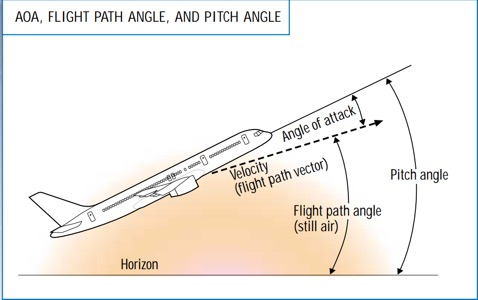Article:
Angle of attack (AOA) is the angle between the oncoming air or relative wind and a reference line on the airplane or wing. Sometimes, the reference line is a line connecting the leading edge and trailing edge at some average point on the wing. Most commercial jet airplanes use the fuselage centerline or longitudinal axis as the reference line. It makes no difference what the reference line is, as long as it is used consistently.

AOA is sometimes confused with pitch angle or flight path angle. Pitch angle (attitude) is the angle between the longitudinal axis (where the airplane is pointed) and the horizon. This angle is displayed on the attitude indicator or artificial horizon.

Flight path angle is defined in two different ways. To the aerodynamicist, it is the angle between the flight path vector (where the airplane is going) and the local atmosphere. To the flight crew, it is normally known as the angle between the flight path vector and the horizon, also known as the climb (or descent) angle. Airmass-referenced and inertial-referenced flight path angles are the same only in still air (i.e., when there is no wind or vertical air movement). For example, in a headwind or sinking air mass, the flight path angle relative to the ground will be less than that referenced to the air. On the newest commercial jet airplanes, this angle can be displayed on the primary flight display and is calculated referenced to the ground (the inertial flight path angle).

AOA is the difference between pitch angle and flight path angle when the flight path angle is referenced to the atmosphere. Because of the relationship of pitch angle, AOA, and flight path angle, an airplane can reach a very high AOA even with the nose below the horizon, if the flight path angle is a steep descent.

Now we ARE in level flight in Gimbal, but the angle I'm using to tilt the plane up and down is pitch, i.e. "the angle between the longitudinal axis (where the airplane is pointed) and the horizon"

This relates to the discussion by @markus about rotation order. What I do in the (rotate about the forward axis by the bank angle, and then about the sideways angle by the pitch angle) matches the definition of pitch, but I don't think it makes sense to describe that as AoA. Pitch is relative to the horizon. AoA is relative to airflow, so when banked it's not the same, and certainly not when climbing or descending. A banked turn could be thought of as "climbing" sideways through the air, so you can have a steep AoA with no pitch (for a bit)

So I think I will drop referring to the upward angle of the jet as the convenient "AoA", and refer to it just as pitch, and "jet pitch" to distinguish it from "Pod (ball) pitch".

#### Mick West

Staff member
Here's a cool little Attitude indicator simulator that shows how pitch is relative to the horizon
http://canvaslib.erau.edu/coa-flight/trainingaids/3dinstruments/attitude.html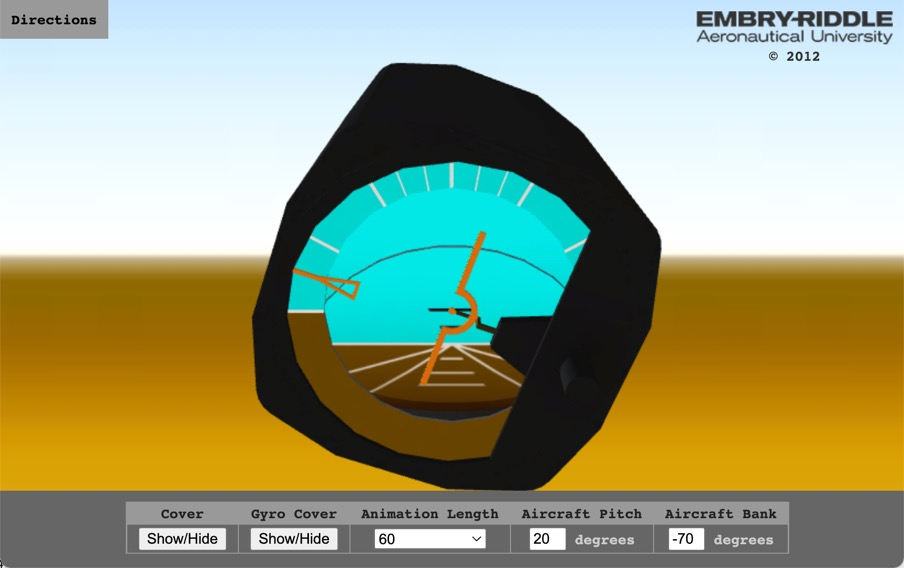Here I'm banked 70° left, pitch is 20° and it's shown as 20° above the horizon, which does not change with bank angle.

Rotate it around and hide the covers, you get a look at the inner workings.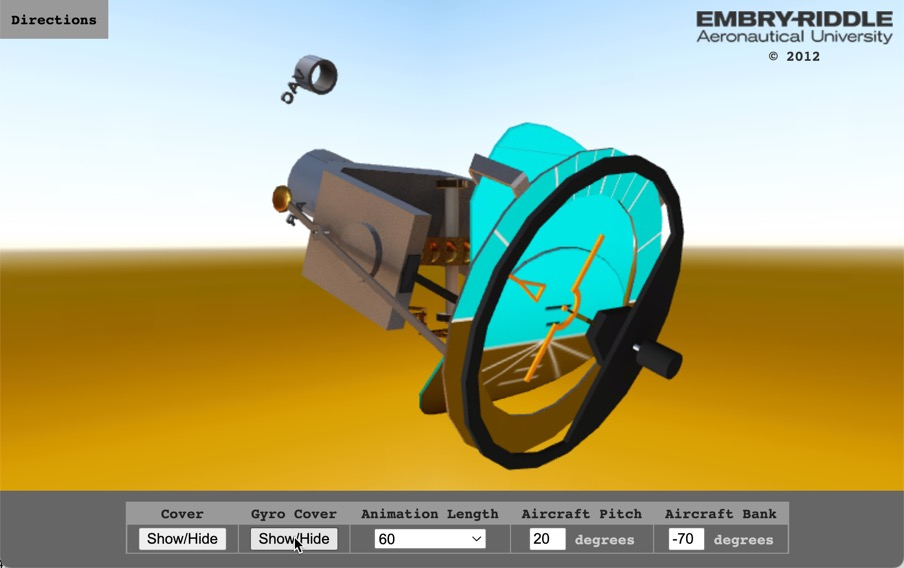#### Mick West

Staff member

A few updates to the sim. I've added the ability to drag the green target around - that represents the physical pointing direction, with a ring around it representing the 5° radius deviation allowed by internal gimballed mirrors.

There have also been a variety of performance improvements, allowing it to work on slower connections and a cleaner display on mobile.

Also, keyboard shortcuts are now displayed on the screen.
https://www.metabunk.org/gimbal/

#### markus

##### Active Member
Some very interesting developments this past weekWith hindsight, it's amusing that the resolution to this issue is pretty much the same as the resolution to the previous issue (compose two rotations in two different axes, and you end up rotating a bit in the remaining axis). Even having that fact in the immediate context this is not obvious.

I don't have much to add except to add some independent confirmation to some of the conclusions.

Before all this I was trying to estimate how the AoA would change as a function of bank angle, so I recorded AoA in DCS in two configurations, one with the jet laden with stores, another after jettisoning everything. I found this:

Heavy:
Code:
``````Bank | AoA
0°   | 6.7°
5°   | 6.7°
15°  | 6.8°
30°  | 7.5°
45°  | 8.9°``````

Light:
Heavy:
Code:
``````Bank | AoA
0°   | 5.6°
5°   | 5.6°
15°  | 5.8°
30°  | 6.4°
45°  | 7.8°``````

I found that in both cases these angles are modeled well in the region of interest by the following empirical relationship (bank in degrees):

AoA(bank) = AoA(0°) + bank^2.5 / 6160

This happens to nearly cancel the effect of projecting the AoA onto the vertical to obtain the pitch, so that in the end the pitch is almost constant throughout the first 31 seconds, which is consistent with the information we get from the cue dot. In the last few seconds with the rapid reduction in bank angle something weird happens. It might be there's a small departure from coordinated flight, but what happens is also described well by a sharp drop in the AoA.

So, assuming that the AoA at 0 bank angle would be 4.5°, we come to the following model: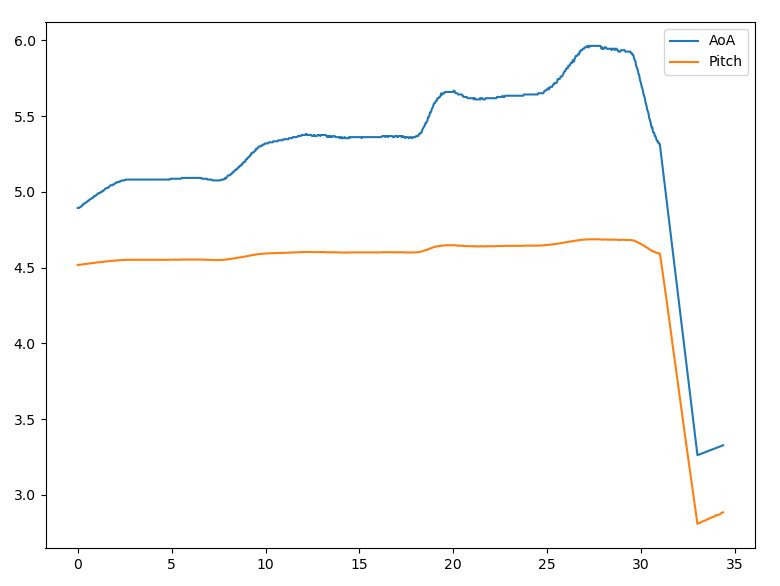which results in the following fit between predicted and actual pip angle:
: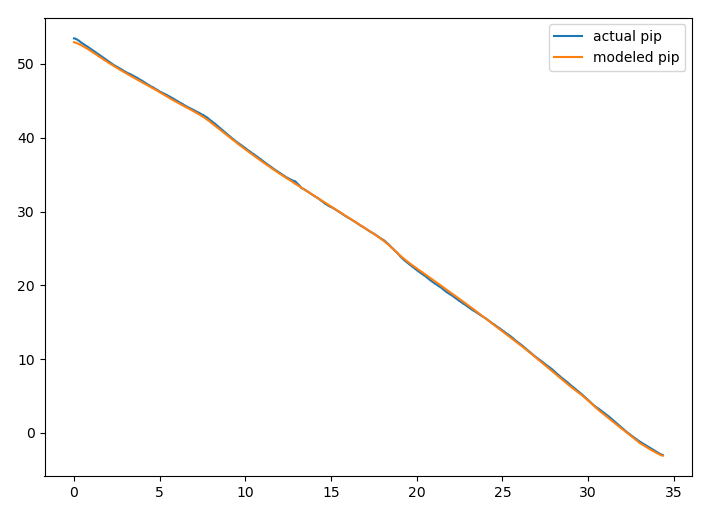(I'm not posting the whole code again because it's gotten quite messy, but since my product is the trajectory of the object in the frame of the jet, it's very simple to calculate: simply grab
`-np.arcsin(y / np.sqrt(x**2 + y**2))` after the bank rotation is applied).

There's bit of uncertainty left in the AoA even with the cue dot indication: we don't know if that indication has some lag, and the cue's trajectory on the screen is not a simple circular arc, which is strange (maybe it's indicating something else, or it's using some crummy approximation for the trigonometric functions). Still, its allowable range is narrowed and it's very nice to be confident that the azimuths really are with respect to the boresight. Great work!

#### Attachments

• aoa.csv
78.7 KB · Views: 45

#### Mick West

Staff member
There's bit of uncertainty left in the AoA even with the cue dot indication: we don't know if that indication has some lag, and the cue's trajectory on the screen is not a simple circular arc
I think it's simply clipping the radius, so it stays on-screen at the top.

Here's an interesting version I just made. Each frame is the brightest pixels in every 10th previous frame. This shows the path of the cue, and also some interesting "horizons" in the clouds as they move at different angles.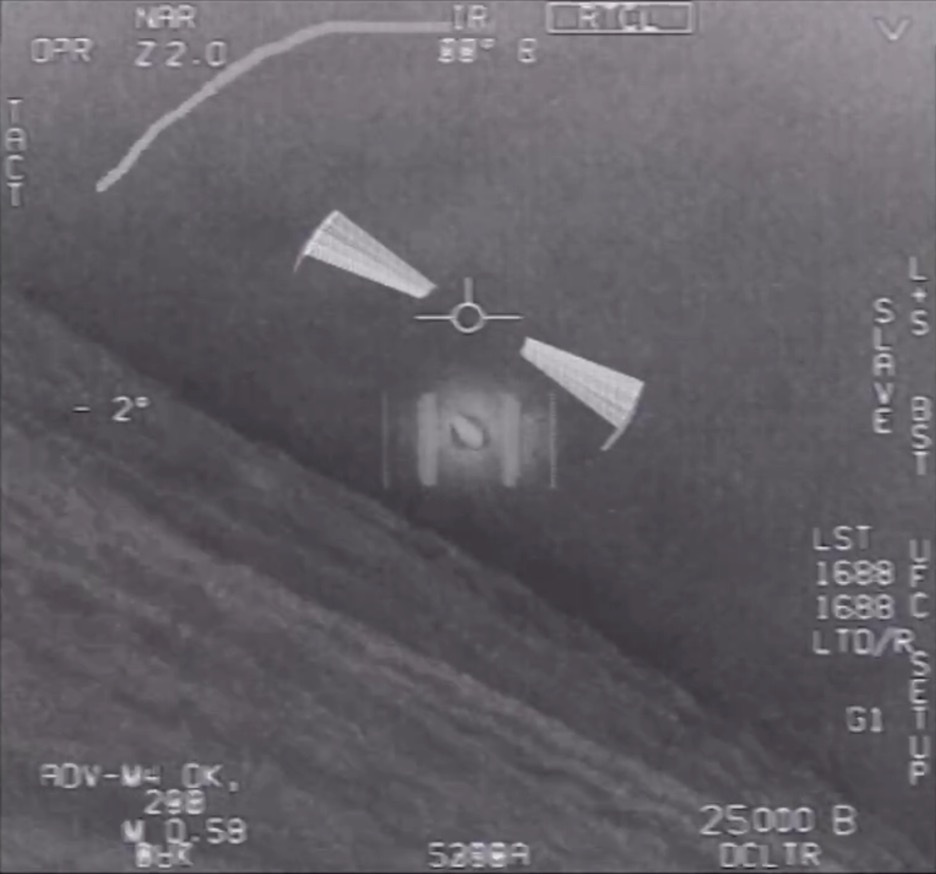#### Leonardo Cuellar

##### Active Member
Heavy:
Code:
``````Bank | AoA
0° | 6.7°
5° | 6.7°
15° | 6.8°
30° | 7.5°
45° | 8.9°``````
Light:
Heavy:
Code:
``````Bank | AoA
0° | 5.6°
5° | 5.6°
15° | 5.8°
30° | 6.4°
45° | 7.8°``````
I found that in both cases these angles are modeled well in the region of interest by the following empirical relationship (bank in degrees):

AoA(bank) = AoA(0°) + bank^2.5 / 6160
Excellent work!
The function that links the angle of attack to the bank angle should be the following (respecting the aerodynamic principles in which up to the stall angle of attack, this angle and the lift coefficient are directly proportional):
AoA = AoA0 * load factor
load factor = 1 / cos (bank angle)

#### dimebag2

##### Active MemberThat's cool. It makes the cloud relief much more apparent. And you finally found the Atlas Rocket!

#### Mick West

Staff member
Before all this I was trying to estimate how the AoA would change as a function of bank angle, so I recorded AoA in DCS in two configurations,Of course, they are the same in straight and level flight. But I wonder if this can be verified as being the same in a level banked turn (what Gimbal and GoFast are doing) in DCS? What instrument gives you the AoA? is this the same as the pitch angle on the artificial horizon?

To add to the naming confusion, "bank" and "roll" do not always refer to the same thing. I found a couple of useful sources. There's Section 3.3. and Appendix C of "Performance of the Jet Transport Airplane" by Trevor M. Young:
https://www.amazon.com/Performance-...&asin=B07ZJNT15C&revisionId=&format=4&depth=1

Section 3.3 (From the Kindle sample linked above) has a simple description.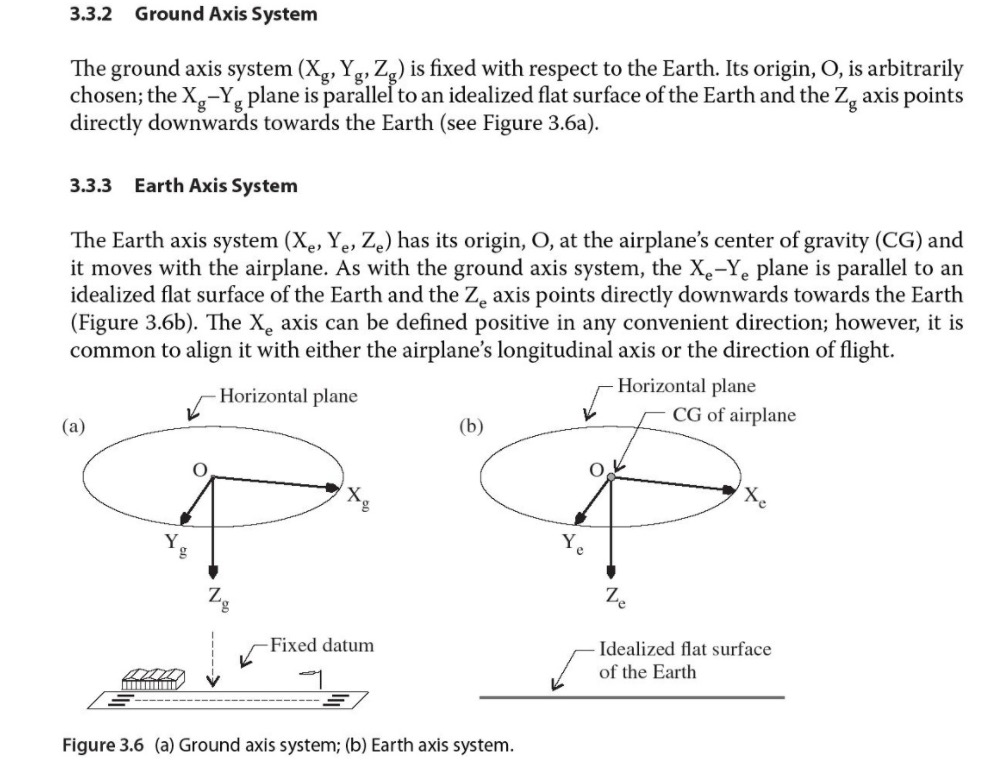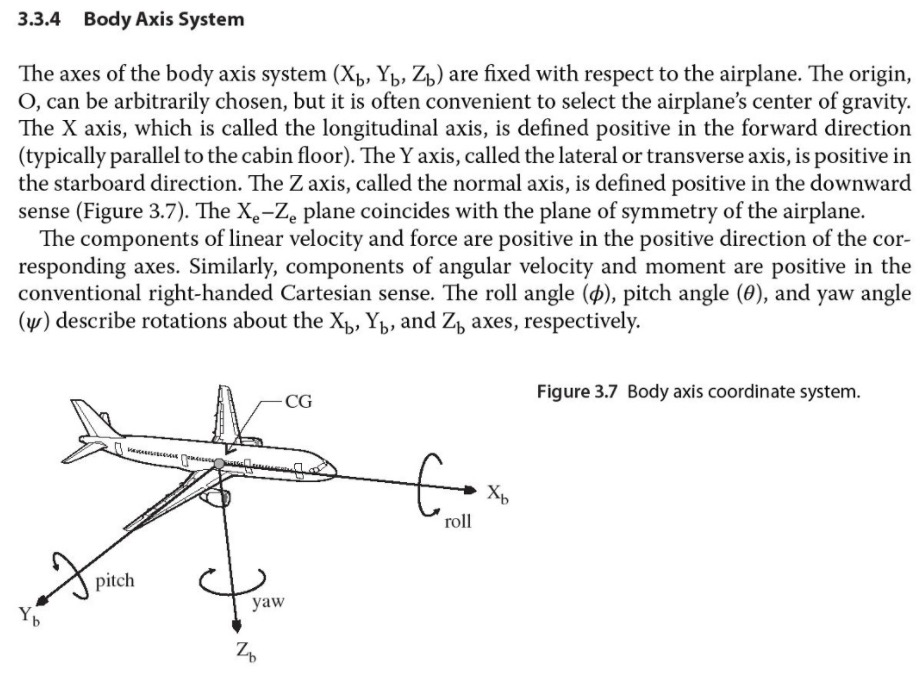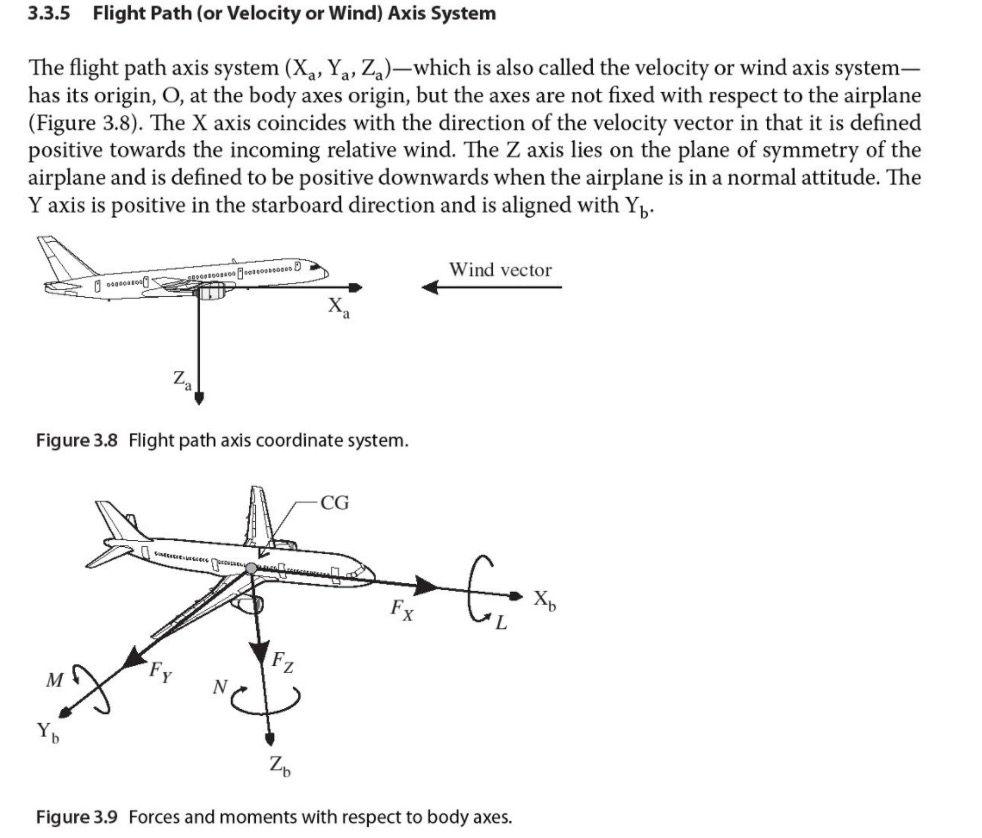The appendix describes the rotations in more detail, and in particular, it describes how "bank angle" is derived from "roll angle" and "pitch"
https://onlinelibrary.wiley.com/doi/pdf/10.1002/9781118534786.app3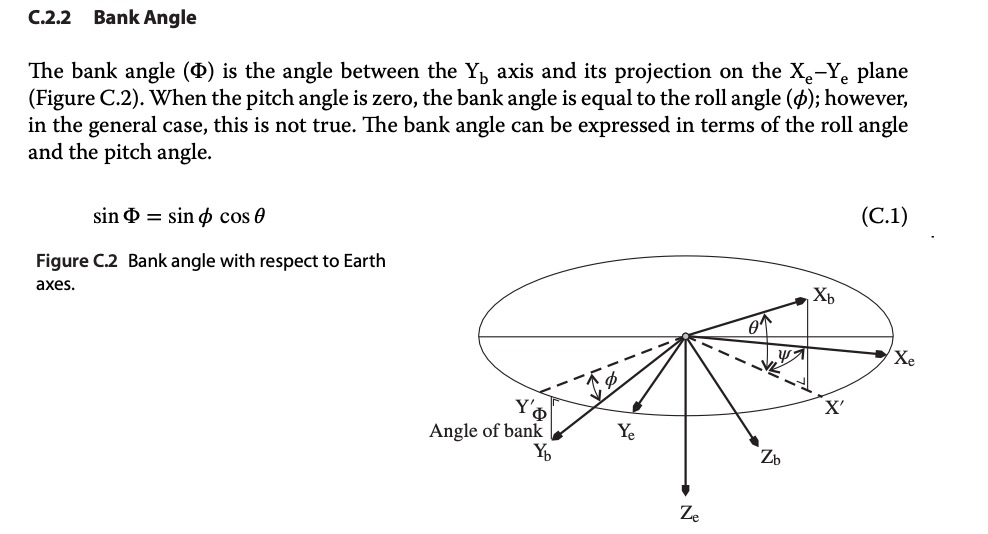sin(Bank) = sin (Roll) * cos(Pitch)
Bank = asin( sin (Roll) * cos(Pitch) )

This does not seem to be particularly significant for the pitch angles under question. At 6° pitch, and 30-degree roll, this gives:
asin(sin(30 degrees)*cos(6 degrees)) in degrees = 29.8 degrees - which is within the margin of error of the screen-scraped roll angle. Cos(6°) is 0.995

However, I'll try to refer to "roll" instead of "bank" - although they can currently be used interchangeably, it's best to be clear where possible.

#### Mick West

Staff member
The function that links the angle of attack to the bank angle should be the following (respecting the aerodynamic principles in which up to the stall angle of attack, this angle and the lift coefficient are directly proportional):
AoA = AoA0 * load factor
load factor = 1 / cos (bank angle)

It sounds like a lot, but in reality, it makes less than 1° of difference in the jet pitch. But I've added it to the sim, so now "Jet Pitch" is the pitch at 0°, which is scaled like:
JavaScript:
``````function jetPitchFromFrame(f=-1) {
if (f == -1) f=par.frame;
var jetPitch = par.jetPitch;
if (par.scaleJetPitch) {
var roll = jetRollFromFrame(f)
}
return jetPitch;
}``````

So I've reduced the default pitch to 3.6° for the best fit with the Cue line, which gives us a very nice fit of under 3° error, with consistent correction rolls. The adjusted pitch varies from 3.9° (at 22° roll) to 4.6° (at 38° roll)

The calculated Cue Az is the cyan line, regular Az is the yellow, the screen-scraped Cue az is the white line.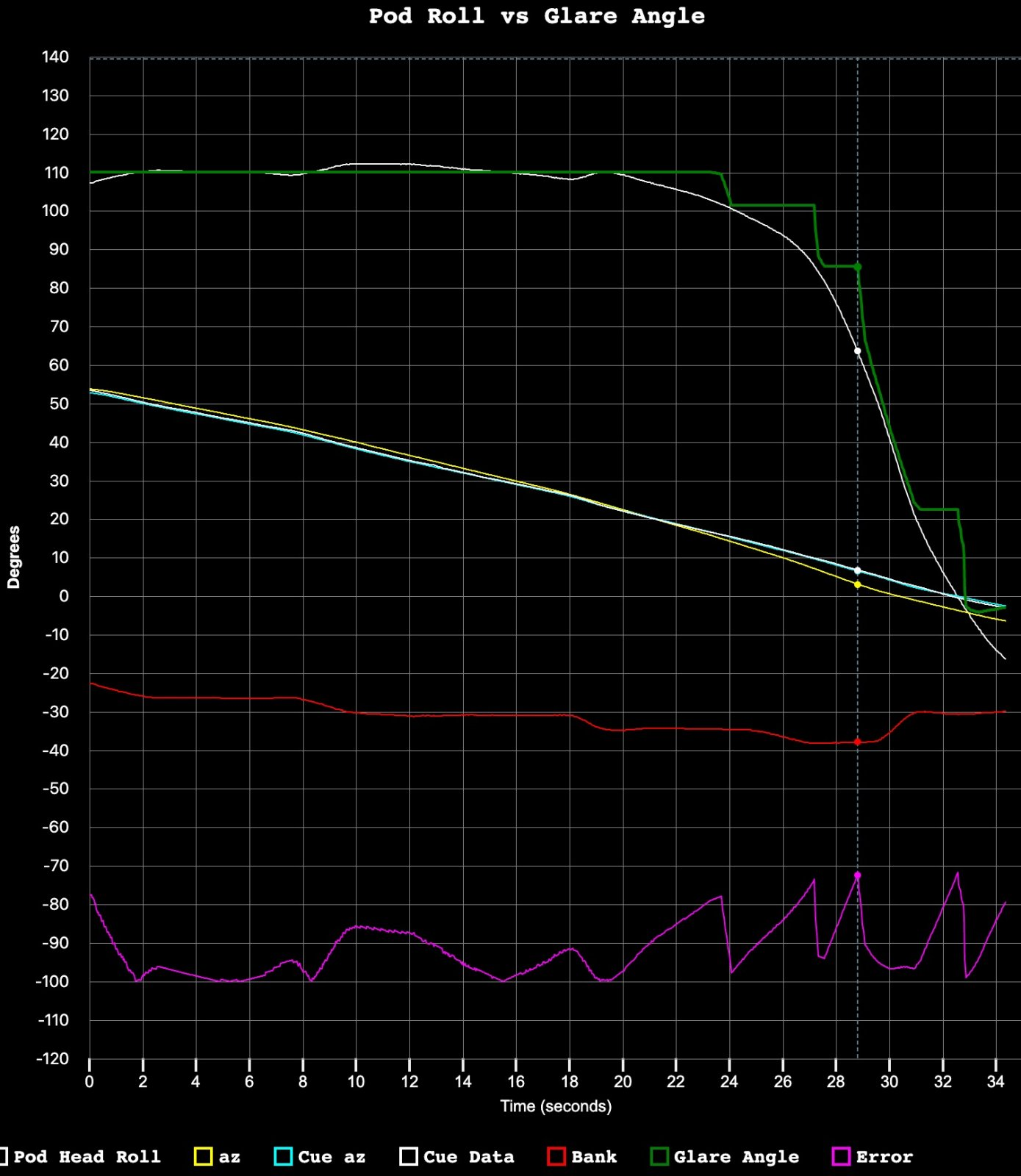The version with a fixed pitch, which is best at 4° to 5° (deviates in different places) , is also a pretty good fit. Here's a constant pitch of 4.6°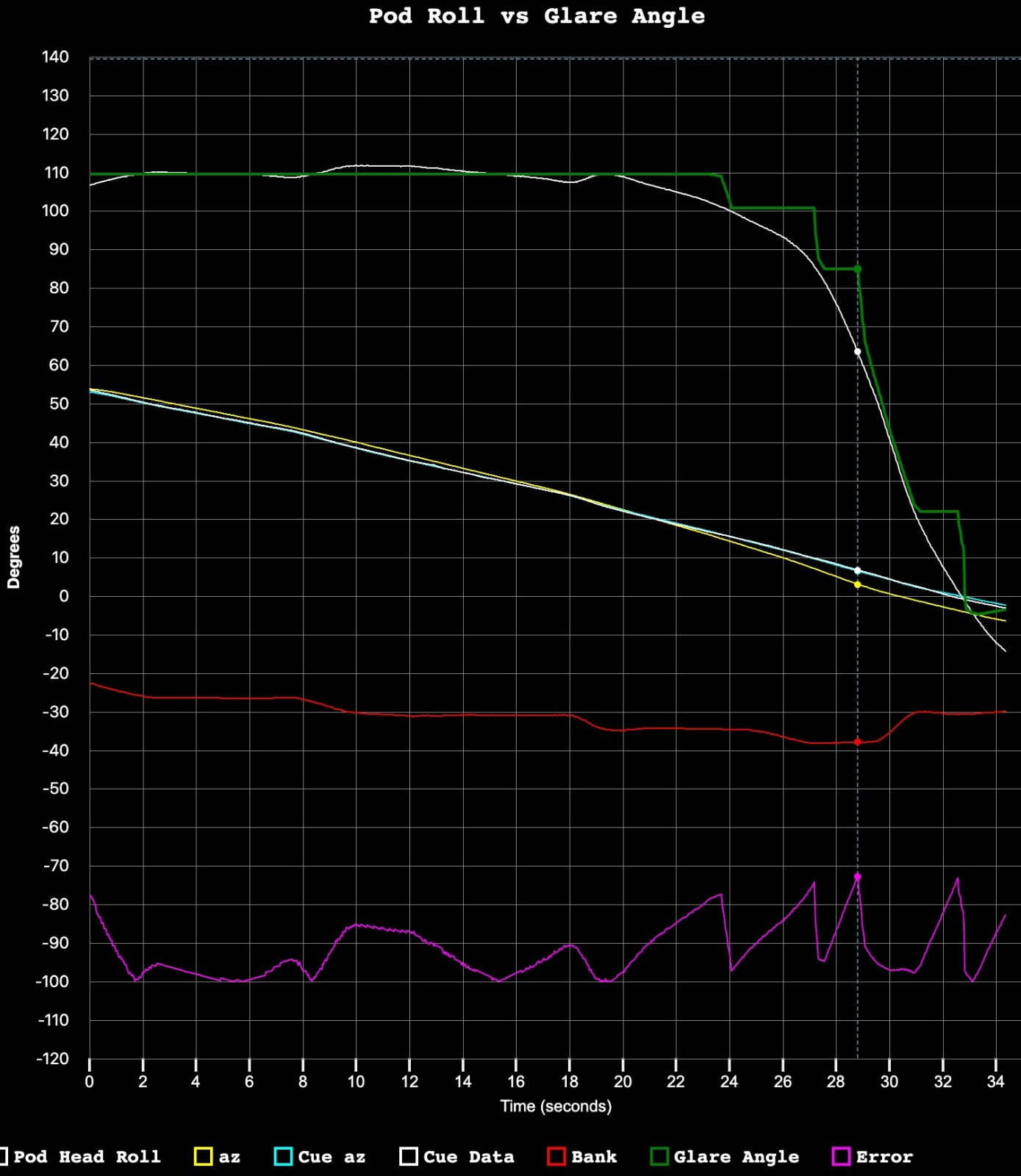The scaled pitch (i.e. nose up more to account for reduced lift in banks) is certainly better, but really just a minor refinement.

This value of 3.6° pitch (AoA, as in level flight), is lower than the values from DCS that @markus found. But it does not seem inconsistent with the values in the F/A-18 ops manual at 25,000 feet when optimizing for range.
https://info.publicintelligence.net/F18-EF-200.pdf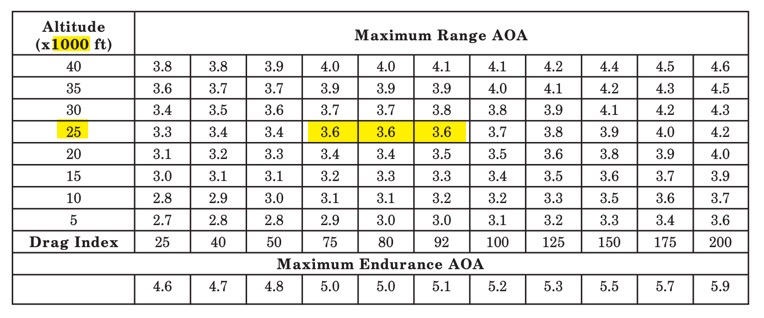#### Leonardo Cuellar

##### Active Member
This value of 3.6° pitch (AoA, as in level flight), is lower than the values from DCS that @markus found. But it does not seem inconsistent with the values in the F/A-18 ops manual at 25,000 feet when optimizing for range.
https://info.publicintelligence.net/F18-EF-200.pdfIn fact this table provides data for two different types of performance. Maximum range or maximum endurance. It would be necessary to know which of the two was applying the fighter to get back on the aircraft carrier. @markus data actually refer to a maximum endurance with minimum drag index, that is, with the fighter completely unloaded.
From the interpolation of the following graphs in the manual, it would seem that mach 0.58 is an optimal maximum endurance speed at 25000ft for a standard mission weight.

Last edited:

#### Leonardo Cuellar

##### Active Member
So, assuming that the AoA at 0 bank angle would be 4.5°, we come to the following model:I don't know how to interpret this graph, where to get the pip congruent to the actual pip, the pitch should drop dramatically by 2 ° in 4 seconds without the flight parameters undergoing significant variations.